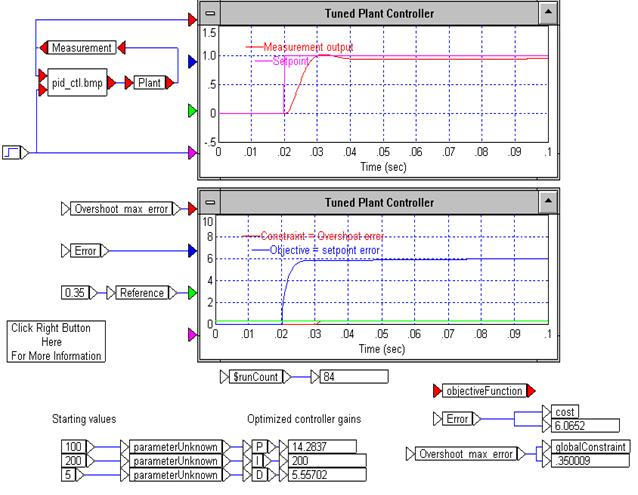### PID tuning problem with three parameters — PIDTUNEZ.VSM

PIDTUNEZ.VSM, an adaptation PID controller tuning diagram (PIDTUNE.VSM) distributed with the Embed base product, describes a PID tuning problem with three parameters and one constraint.

The PID controller has three decision variables:

A proportional gain (pg)

An integral gain (ig)

A derivative gain (dg)

Once these variables are set, a simulation run produces the resulting output signal (s). Assuming that a desired response (r) is postulated and that the functions r and s are defined on the interval [0, .1], one measure of the fidelity of s to r is to compute the integrated squared error between these two functions. This integral is the cost function. Notice that this function depends on s, which depends on both the input signal (which is assumed to be given and fixed) and the PID variables. Thus, choosing the decision variables optimally would be equivalent to choosing the pg, ig, and the dg to minimize the integrated squared error between the response and the resulting output signal.

Taking this example one step further, it is easy to see that certain values for the gains are not reasonable. Thus, you can restrict the decision variables by:

10  ≤ pg ≤ 1000

200  ≤ ig  ≤  200

0  ≤ dg ≤  100

You set these upper and lower bounds in the Properties dialog box for the parameterUnknown blocks. Notice that the integral gain is fixed at 200.

Finally, to prevent the output signal (s) from overshooting the upper value of r(t) by a certain amount, you can set the globalConstraint as:

max {m(t) : 0 ≤ t ≤ .1}  ≤ .35

where

m(t) := 20 (s(t) - r(t))

In this problem, this has the effect that s(t) ≤ 1+(.35)/20.

If you start at pg = 100, ig = 200, and dg = 5, the value of the objective function is 6.02. The overshoot error is 1.41, which exceeds the maximum allowed overshoot of 0.35. Thus, the problem is infeasible at the starting point. Embed converged after 84 simulation runs with the objective function at 6.06 and the overshoot error at its upper limit of 0.35.

The PIDTUNEZ diagram shows the final and optimal values of the gains.